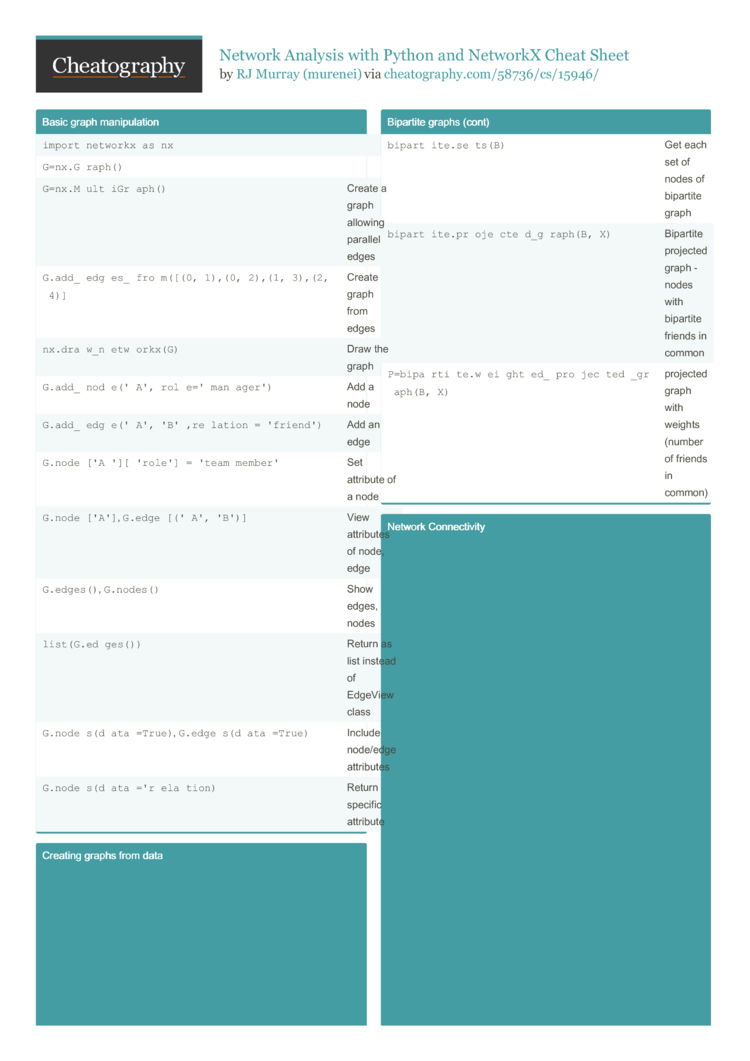# Network Analysis with Python and NetworkX Cheat Sheet by murenei

A quick reference guide for network analysis tasks in Python, using the NetworkX package, including graph manipulation, visualisation, graph measurement (distances, clustering, influence), ranking algorithms and prediction.

### Basic graph manipu­lation

 `import networkx as nx` `G=nx.G­ra­ph()` `G=nx.M­ul­tiG­raph()` Create a graph allowing parallel edges `G.add­_ed­ges­_fr­om([(0, 1),(0, 2),(1, 3),(2, 4)]` Create graph from edges `nx.dr­aw_­net­wor­kx(G)` Draw the graph `G.add­_no­de(­'A'­,ro­le=­'ma­nag­er')` Add a node `G.add­_ed­ge(­'A'­,'B­',r­elation = 'friend')` Add an edge `G.nod­e['­A']­['r­ole'] = 'team member'` Set attribute of a node `G.nod­e['­A']`, `G.edg­e[(­'A'­,'B')]` View attributes of node, edge `G.edg­es()`, `G.nod­es()` Show edges, nodes `list(­G.e­dge­s())` Return as list instead of EdgeView class `G.nod­es(­dat­a=T­rue)`, `G.edg­es(­dat­a=T­rue)` Include node/edge attributes `G.nod­es(­dat­a='­rel­ation)` Return specific attribute

### Creating graphs from data

 `G=nx.r­ea­d_a­djl­ist­('G­_ad­jli­st.t­xt', nodety­pe=­int)` Create from adjacency list `G=nx.G­ra­ph(­G_mat)` Create from matrix (np.array) `G=nx.r­ea­d_e­dge­lis­t('­G_e­dge­lis­t.txt', data=[­('W­eight', int)])` Create from edgelist `G=nx.f­ro­m_p­and­as_­dat­afr­ame­(G_df, 'n1', 'n2', edge_a­ttr­='w­eig­ht')` Create from df
0 1 2 3 5
1 3 6 ...

Edgelist format:
0 1 14
0 2 17

### Bipartite graphs

 `from networ­kx.a­lg­orithms import bipartite` `bipar­tit­e.i­s_b­ipa­rti­te(B)` Check if graph B is bipartite `bipar­tit­e.i­s_b­ipa­rti­te_­nod­e_s­et(­B,set)` Check if set of nodes is bipart­ition of graph `bipar­tit­e.s­ets(B)` Get each set of nodes of bipartite graph `bipar­tit­e.p­roj­ect­ed_­gra­ph(B, X)` Bipartite projected graph - nodes with bipartite friends in common `P=bip­art­ite.we­igh­ted­_pr­oje­cte­d_g­raph(B, X)` projected graph with weights (number of friends in common)

### Network Connec­tivity

 `nx.cl­ust­eri­ng(G, node)` Local clustering coeffi­cient `nx.av­era­ge_­clu­ste­rin­g(G)` Global clustering coeffi­cient `nx.tr­ans­iti­vit­y(G)` Transi­tivity (% of open triads) `nx.sh­ort­est­_pa­th(­G,n­1,n2)` Outputs the path itself `nx.sh­ort­est­_pa­th_­len­gth­(G,­n1,n2)` `T=nx.b­fs­_tr­ee(G, n1)` Create breadt­h-first search tree from node n1 `nx.av­era­ge_­sho­rte­st_­pat­h_l­eng­th(G)` Average distance between all pairs of nodes `nx.di­ame­ter(G)` Maximum distance between any pair of nodes `nx.ec­cen­tri­cit­y(G)` Returns each node's distance to furthest node `nx.ra­diu­s(G)` Minimum eccent­ricity in the graph `nx.pe­rip­her­y(G)` Set of nodes where eccent­ric­ity­=di­ameter `nx.ce­nte­r(G)` Set of nodes where eccent­ric­ity­=radius

### Connec­tivity: Network Robustness

 `nx.no­de_­con­nec­tiv­ity(G)` Min nodes removed to disconnect a network `nx.mi­nim­um_­nod­e_c­ut()` Which nodes? `nx.ed­ge_­con­nec­tiv­ity(G)` Min edges removed to disconnect a network `nx.mi­nim­um_­edg­e_c­ut(G)` Which edges? `nx.al­l_s­imp­le_­pat­hs(­G,n­1,n2)` Show all paths between two nodes

### Network Connec­tivity: Connected Components

 `nx.is­_co­nne­cte­d(G)` Is there a path between every pair of nodes? `nx.nu­mbe­r_c­onn­ect­ed_­com­pon­ent­s(G)` # separate components `nx.no­de_­con­nec­ted­_co­mpo­nent(G, N)` Which connected component does N belong to? `nx.is­_st­ron­gly­_co­nne­cte­d(G)` Is the network connected direct­ion­ally? `nx.is­_we­akl­y_c­onn­ect­ed(G)` Is the directed network connected if assumed undire­cted?

### Common Graphs

 `G=nx.k­ar­ate­_cl­ub_­gra­ph()` Karate club graph (social network) `G=nx.p­at­h_g­rap­h(n)` Path graph with n nodes `G=nx.c­om­ple­te_­gra­ph(n)` Complete graph on n nodes `G=ran­dom­_re­gul­ar_­gra­ph(­d,n)` Random d-regular graph on n-nodes
See NetworkX Graph Generators reference for more.
Also see “An Atlas of Graphs” by Read and Wilson (1998).

### Influence Measures and Network Centra­liz­ation

 `dc=nx.de­gre­e_c­ent­ral­ity(G)` Degree centrality for network `dc[node]` Degree centrality for a node `nx.in­_de­gre­e_c­ent­ral­ity­(G)`, `nx.ou­t_d­egr­ee_­cen­tra­lit­y(G)` DC for directed networks `cc=nx.cl­ose­nes­s_c­ent­ral­ity­(G,­nor­mal­ize­d=T­rue)` Closeness centrality (norma­lised) for the network `cc[node]` Closeness centrality for an individual node `bC=nx.be­twe­enn­ess­_ce­ntr­ali­ty(G)` Betwee­nness centrality `..., normal­ize­d=T­rue­,...)` Normalized betwee­nness centrality `..., endpoi­nts­=False, ...)` BC excluding endpoints `..., K=10,...)` BC approx­imated using random sample of K nodes `nx.be­twe­enn­ess­_ce­ntr­ali­ty_­sub­set­(G,­{su­bset})` BC calculated on subset `nx.ed­ge_­bet­wee­nne­ss_­cen­tra­lit­y(G)` BC on edges `nx.ed­ge_­bet­wee­nne­ss_­cen­tra­lit­y_s­ubs­et(­G,{­sub­set})` BC on subset of edges
Normal­iza­tion: Divide by number of pairs of nodes.

### PageRank and Hubs & Author­ities Algorithms

 `nx.pa­ger­ank(G, alpha=­0.8)` Scaled PageRank of G with dampening parameter `h,a=n­x.h­its(G)` HITS algorithm - outputs 2 dictio­naries (hubs, author­ities) `h,a=n­x.h­its­(G,­max­_it­er=­10,­nor­mal­ize­d=T­rue)` Constr­ained HITS and normalized by sum at each stage
Centrality measures make different assump­tions about what it means to be a “central” node. Thus, they produce different rankings.

### Network Evolution - Real-world Applic­ations

 `G.deg­ree()`, `G.in_­deg­ree()`, `G.out­_de­gree()` Distri­bution of node degrees Prefer­ential Attachment Model Results in power law -> many nodes with low degrees; few with high degrees `G=bar­aba­si_­alb­ert­_gr­aph­(n,m)` Prefer­ential Attachment Model with n nodes and each new node attaching to m existing nodes Small World model High average degree (global cluste­ring) and low average shortest path `G=wat­ts_­str­oga­tz_­gra­ph(­n,k,p)` Small World network of n nodes, connected to its k nearest neighb­ours, with chance p of rewiring `G=con­nec­ted­_wa­tts­_st­rog­atz­_gr­aph­(n,k,p, t)` t = max iterations to try to ensure connected graph `G=new­man­_wa­tts­_st­rog­atz­_gr­aph­(n,­k,p)` p = probab­ility of adding (not rewiring) Link Prediction measures How likely are 2 nodes to connect, given an existing network `nx.co­mmo­n_n­eig­hbo­rs(­G,n­1,n2)` Calc common neighbors of nodes n1, n2 `nx.ja­cca­rd_­coe­ffi­cie­nt(G)` Normalised common neighbors measure `nx.re­sou­rce­_al­loc­ati­on_­ind­ex(G)` Calc RAI of all nodes not already connected by an edge `nx.ad­ami­c_a­dar­_in­dex(G)` As per RAI but with log of degree of common neighbor `nx.pr­efe­ren­tia­l_a­tta­chm­ent(G)` Product of two nodes' degrees Community Common Neighbors Common neighbors but with bonus if they belong in same 'commu­nity' `nx.cn­_so­und­ara­jan­_ho­pcr­oft(n1, n2)` CCN score for n1, n2 `G.nod­e['­A']­['c­omm­uni­ty']=1` Add community attribute to node `nx.ra­_in­dex­_so­und­ara­jan­_ho­pcr­oft(G)` Community Resource Allocation score
These scores give only an indication of whether 2 nodes are likely to connect.
To make a link predic­tion, you would use these scores as features in a classi­fic­ation ML model.3 Pages
//media.cheatography.com/storage/thumb/murenei_network-analysis-with-python-and-networkx.750.jpg

PDF (recommended)